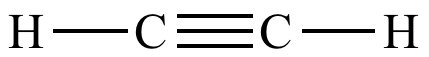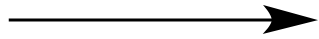Illustrated Glossary of Organic Chemistry

One equivalent: In reaction stoichiometry, the amount of one substance that reacts with one mole of another substance. This will often (but not always) be a 1:1 mole ratio.In this SN2 reaction, one mole of CH3Cl reacts with one mole of NaI to produce one mole of CH3I and one mole of NaCl. One equivalent of CH3Cl reacts with one equivalent of NaI.2 eq. H2PtIn this catalytic hydrogenation reaction, one mole of acetylene reacts with two moles of H2 to produce one mole of ethane.1 eq. H2Pt1 eq. H2PtReaction of acetylene with one equivalent of H2 gives ethylene; reaction of acetylene with two equivalents (2 eq.) of H2 gives ethane.